# HSPT Math : Geometry

## Example Questions

← Previous 1 3 4 5 6 7 8 9 25 26

### Example Question #1 : How To Find The Area Of A Figure

What is the area of a circle with a radius of 10?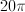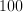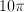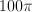Explanation:

The formula for the area of a circle is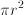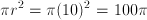### Example Question #1 : How To Find The Area Of A Figure

What is the area of a circle with a radius of 7?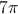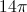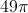Explanation:

To find the area of a circle you must plug the radius intoin the following equation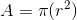In this case the radius is 7 so we plug it intoto get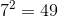We then multiply it by pi to get our answer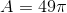### Example Question #1 : How To Find The Area Of A Figure

What is the area of a square with a side length of 8?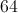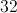Explanation:

To solve this question you must know the formula for the area of a rectangle.

The formula is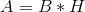In this case the rectangle is a square so we can plug in the side length for both base and height to yield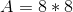Perform the multiplication to arrive at the answer of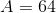### Example Question #2 : How To Find The Area Of A Figure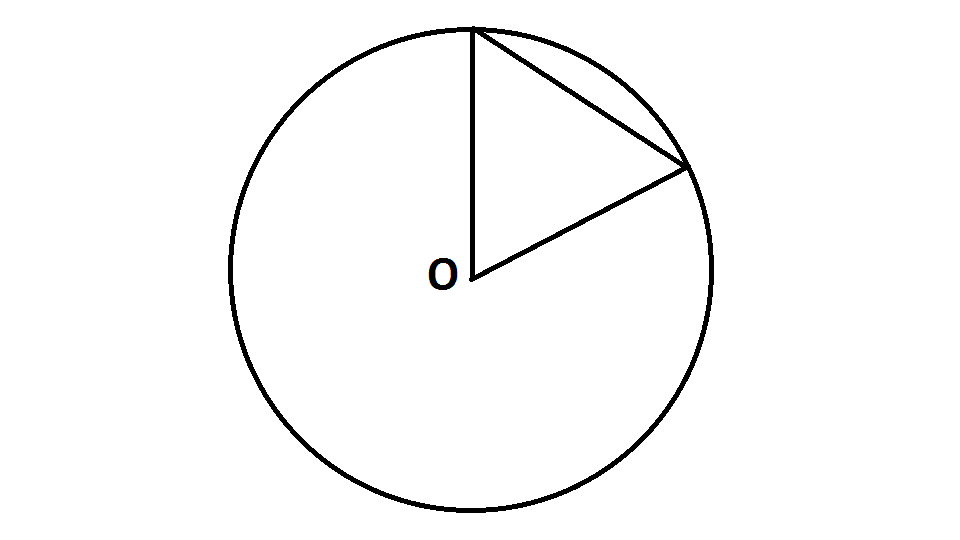Refer to the above diagram, which shows an equilateral triangle with one vertex at the center of a circle and two vertices on the circle.

What percent of the circle (nearest whole number) is covered by the triangle?

10%

12%

18%

It is impossible to tell without knowing the radius.

14%

14%

Explanation:

Letbe the radius of the circle. The area of the circle is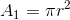is also the sidelength of the equilateral triangle. The area of the triangle is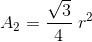The percent of the circle covered by the triangle is: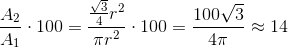### Example Question #1 : Geometry

Julie wants to seed her rectangular lawn, which measures 265 feet by 215 feet. The grass seed she wants to use gets 400 square feet of coverage to the pound; a fifty-pound bag sells for $66.00, and a ten-pound bag sells for$20.00. What is the least amount of money Julie should expect to spend on grass seed?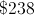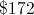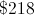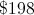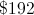Explanation:

The area of Julie's lawn is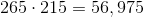square feet. The amount of grass seed she needs is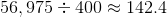pounds. This requires three fifty-pound bags, the most economical option since it is cheaper to buy a fifty-pound bag for $66 than five ten-pound bags for$100.00. Julie will spend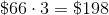### Example Question #5 : How To Find The Area Of A Rectangle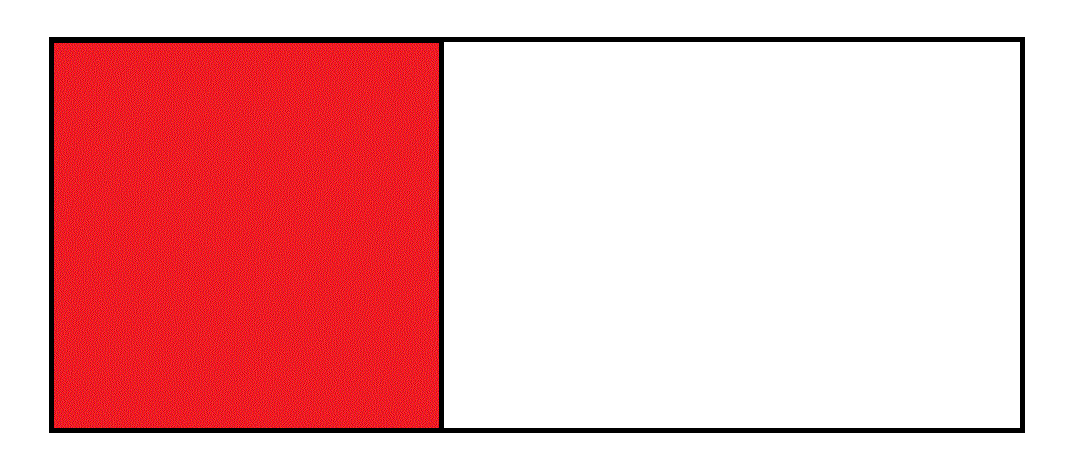Above is a figure that comprises a red square and a white rectangle. The ratio of the length of the white rectangle to the sidelength of the square is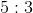. What percent of the entire figure is red?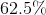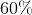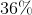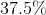Explanation:

To make this easier, we will assume that the rectangle has length 5 and the square has sidelength 3. Then the area of the entire figure is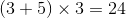,

and the area of the square is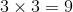The square, therefore, takes up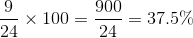of the entire figure.

### Example Question #2 : How To Find The Area Of A Rectangle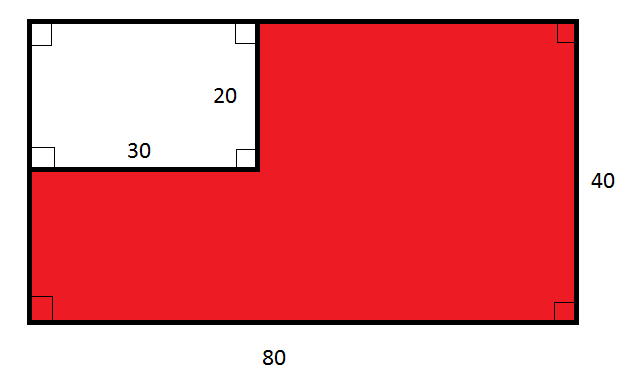Note: Figure NOT drawn to scale.

What percent of the above figure is white?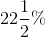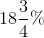Explanation:

The large rectangle has length 80 and width 40, and, consequently, area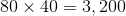.

The white region is a rectangle with length 30 and width 20, and, consequently, area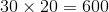.

The white region is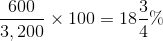of the large rectangle.

### Example Question #1 : How To Find The Area Of A Figure

A square is 9 feet long on each side.  How many smaller squares, each 3 feet on a side can be cut out of the larger square?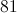Explanation:

Each side can be divided into three 3-foot sections.  This gives a total ofsquares.  Another way of looking at the problem is that the total area of the large square is 81 and each smaller square has an area of 9.  Dividing 81 by 9 gives the correct answer.

### Example Question #1 : How To Find The Area Of A Circle

Assume π = 3.14

A man would like to put a circular whirlpool in his backyard. He would like the whirlpool to be six feet wide. His backyard is 8 feet long by 7 feet wide. By state regulation, in order to put a whirlpool in a backyard space, the space must be 1.5 times bigger than the pool. Can the man legally install the whirlpool?

No, because the area of the backyard is smaller than the area of the whirlpool.

No, because the area of the backyard is 30 square feet and therefore the whirlpool is too big to meet the legal requirement.

Yes, because the area of the whirlpool is 28.26 square feet and 1.5 times its area would be less than the area of the backyard.

Yes, because the area of the whirlpool is 18.84 square feet and 1.5 times its area would be less than the area of the backyard.

No, because the area of the whirlpool is 42.39 square feet and 1.5 times its area would be greater than the area of the backyard.

Yes, because the area of the whirlpool is 28.26 square feet and 1.5 times its area would be less than the area of the backyard.

Explanation:

If you answered that the whirlpool’s area is 18.84 feet and therefore fits, you are incorrect because 18.84 is the circumference of the whirlpool, not the area.

If you answered that the area of the whirlpool is 56.52 feet, you multiplied the area of the whirlpool by 1.5 and assumed that that was the correct area, not the legal limit.

If you answered that the area of the backyard was smaller than the area of the whirlpool, you did not calculate area correctly.

And if you thought the area of the backyard was 30 feet, you found the perimeter of the backyard, not the area.

The correct answer is that the area of the whirlpool is 28.26 feet and, when multiplied by 1.5 = 42.39, which is smaller than the area of the backyard, which is 56 square feet.

### Example Question #1 : Plane Geometry

A square with a side length of 4 inches is inscribed in a circle, as shown below. What is the area of the unshaded region inside of the circle, in square inches?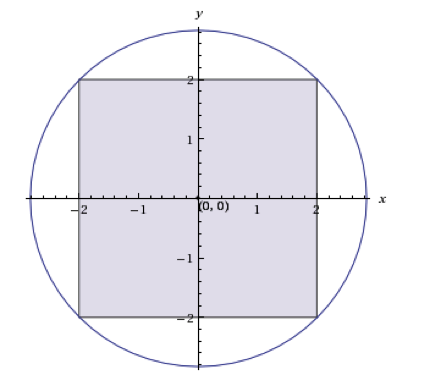8π - 16

2π-4

8π-8

8π-4

4π-4